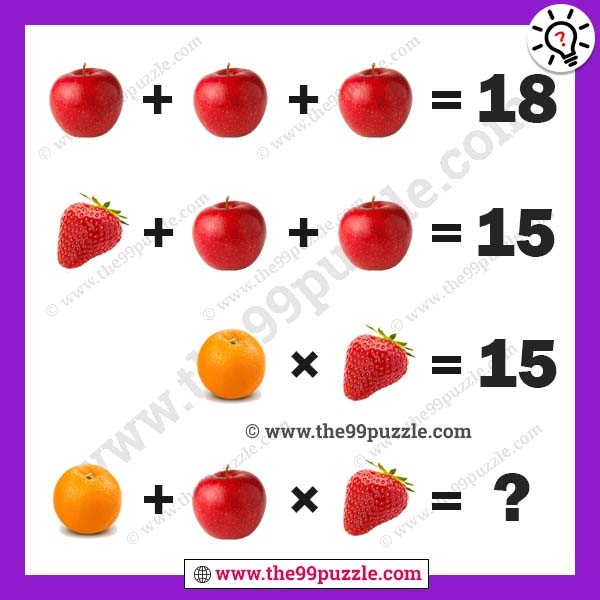# Fruits pictures math equation for teens – Puzz170

Fruits pictures math equation for teens. Math logic brain teaser for genius minds. These picture puzzles are challenging for people. Can you calculate the math equation picture puzzle?###### Explanation:

Apple + Apple + Apple = 18

Strawberry + Apple + Apple = 15

Orange × Strawberry = 15

Orange + Apple × Strawberry = ?

6 + 6 + 6 = 18

3 + 6 + 6 = 15

5 × 3 = 15

5 + 6 × 3 = 23